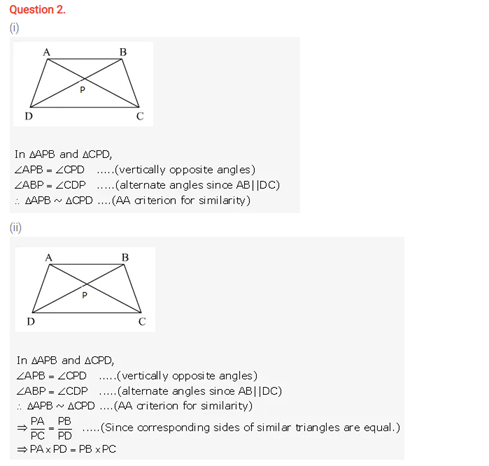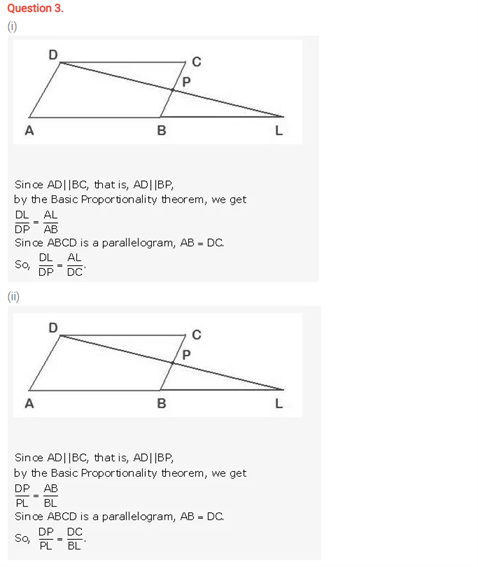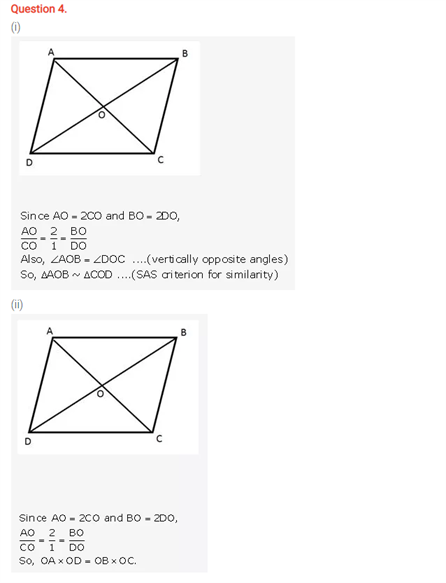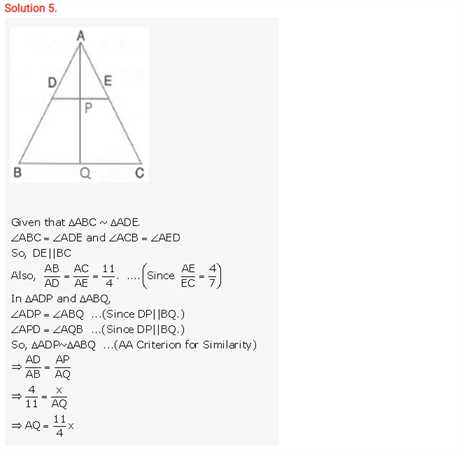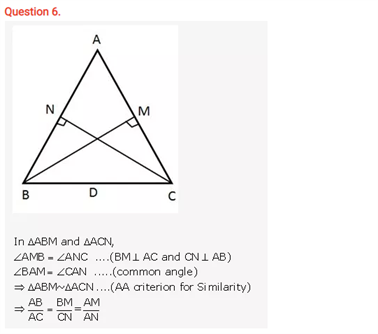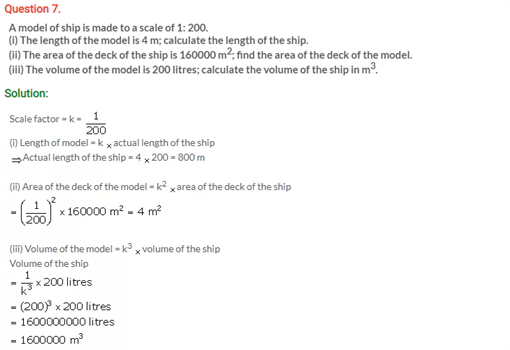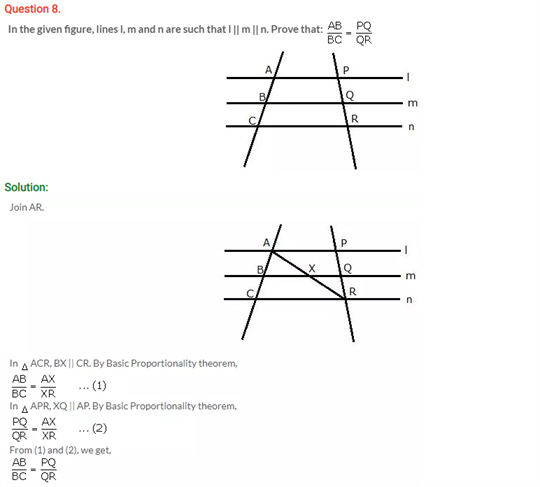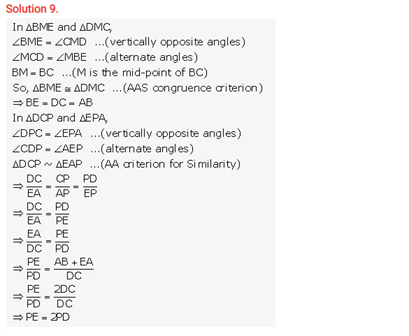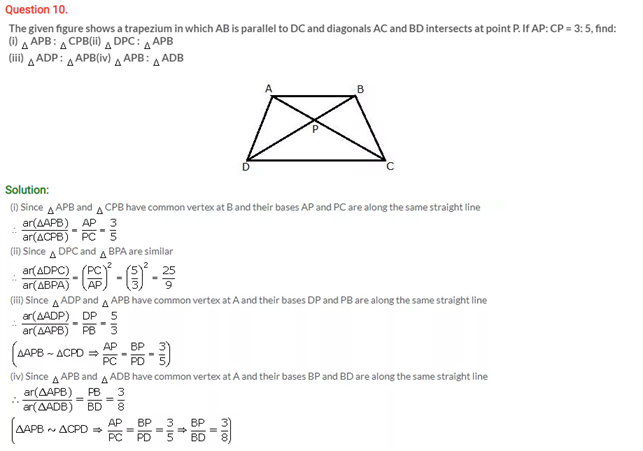# Selina Concise Maths Solution for ICSE Class 10 Chapter 15 Similarity (With Applications to Maps and Models)

## Selina Class 10 Maths Solutions for Chapter 15

Solving the solutions on Similarity from the ICSE Class 10 Maths Selina Solutions will ensure a better grasp of this chapter. The problems of this chapter can be viewed below. From a geometrical point of view, knowing the congruent and using the similar shapes will ease up the calculations and the design work. We will find a mathematical meaning of the terms similar and congruent. If two figures are said to be congruent then, if you move or rotate the shape of one, it will still be congruent to the shape of the other. These questions below belong to the above concept.

Students can view the Class 10 Selina Maths ICSE Solutions Chapter 15 Similarity below: